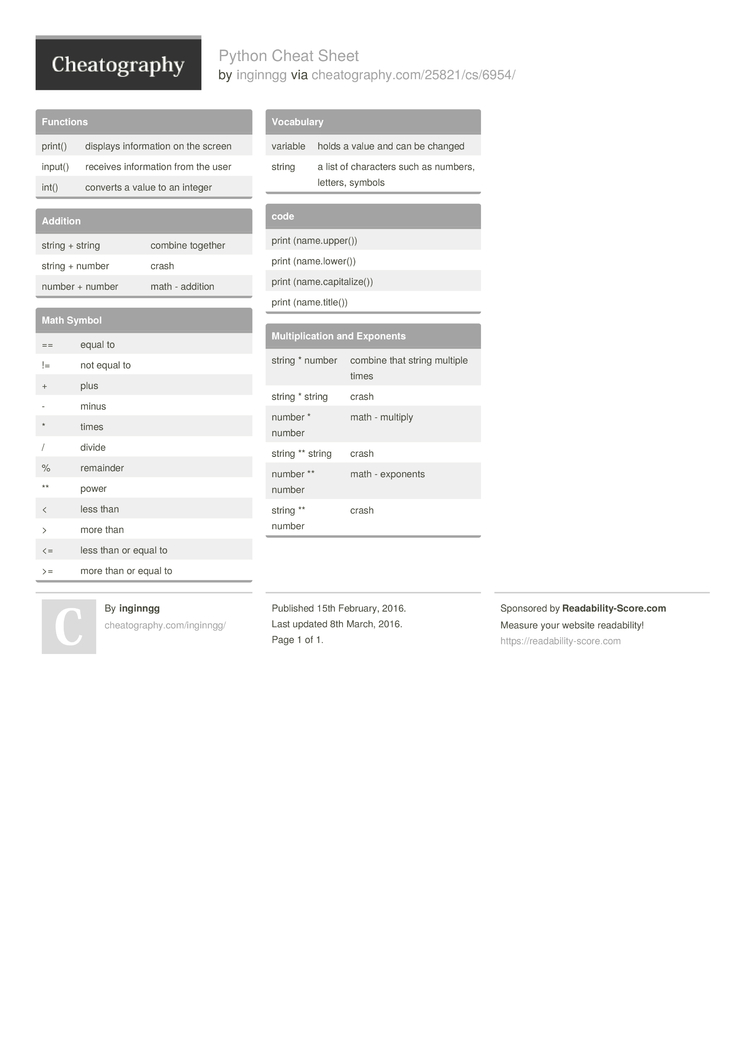Cheatography

# Python Cheat Sheet by inginngg

 string + string combine together string + number crash number + number math - addition

### Math Symbol

 == equal to != not equal to + plus - minus * times / divide % remainder ** power < less than > more than <= less than or equal to >= more than or equal to

### Functions

 print() displays inform­­ation on the screen input() receives inform­­ation from the user int() converts a value to an integer

### Vocabulary

 variable holds a value and can be changed string a list of characters such as numbers, letters, symbols

### code

 print (name.u­pp­er()) print (name.l­ow­er()) print (name.c­ap­ita­lize()) print (name.t­it­le())

### Multip­­li­c­ation and Exponents

 string * number combine that string multiple times string * string crash number * number math - multiply string ** string crash number ** number math - exponents string ** number crash1 Page
//media.cheatography.com/storage/thumb/inginngg_python.750.jpg

PDF (recommended)